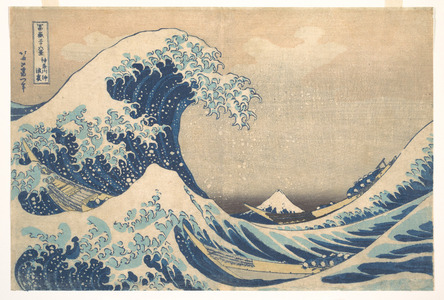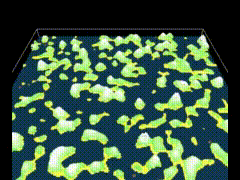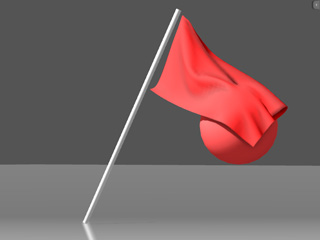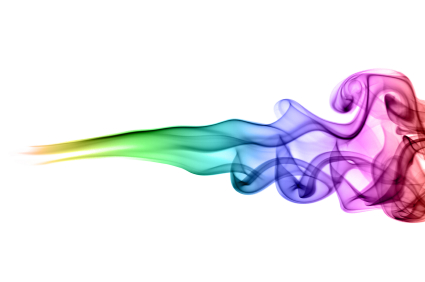# Classical elements in NetLogo: Water

Última modificación: 3 de Diciembre de 2016, y ha tenido 1435 vistas

Etiquetas utilizadas: || || || || ||After Earth, and to continue with the simulation of Classical Elements in NetLogo, in this post we will give some simple, but very graphical and good looking, models to simulate the behaviour of water. Water is a liquid, a type of fluid... right?

But, what is a fluid? A fluid is any substance that flows, and can take the shape of its container, and does not resist deformation, that is, it slides when receiving some pressure. Usually, the terms fluid and liquid are used interchangeable, but technically the term fluid can refer to either a liquid or a gas. The difference between them is that a gas it is not (so) affected by the gravity and fills its container (if any) completely, whereas a liquid under gravity has a "free surface" (the top) whose shape does not depend on its container. Consequently, in the computer simulations to visualize a liquid, only this free surface is computed and rendered, as the other parts are occluded by the container... and this will be our case. The distinction between liquids and gasses can determine how to model the fluid, but from a physical and mathematical point of view, both obey the same basic laws and their differences can be given by the values of some parameters that model them.Usual modelling of physical objects follows an increasing chain of particularities, from more simple to more complex we can find:

1. Particles: points with position, mass, velocity ... but no size or shape. Usually are modelled using basic linear laws.2. Rigid bodies: add shape and orientation to position, mass, and velocity. They are still easy to simulate under isolation, and the hard tasks come from detecting and responding to collisions with the environment. A set of rigid bodies are usually harder to simulate, because all the elements in the set can continuously collides between them.3. Articulated rigid bodies: are connected networks of rigid bodies. The behaviour is similar to the set of rigid bodies, but the collisions between them are restricted to a limited options (usually called constraints of the system).4. Deformable bodies: can change shape but not with totally freedom, they keep their connectedness and adjacency of various points on the body. Examples can be: chains in 1D, clothes in 2D, or soft bodies in 3D.5. Fluids: if you see them as one body, their shape/topology can change. But you can see them as a set of free particles with continuous interaction between them and the environment. Because fluids take the shape of their container, they are always in collision with everything around them, including the fluid itself, hence a collision with one part of the fluid effectively means that the whole body of fluid must respond. They require a huge amount of calculations in order to predict the dynamics of the whole system, and it is very common to try to reduce the complexity by statistical calculations.In this post we will simplify so much the assumptions that the model we will obtain only will be useful to simulate liquids under some conditions, but not gasses. You can find very realistic and nice simulation of different fluids behaviours under several and more general assumptions, but here we will give only a fast and simple way to obtain a behaviour that we visually recognize as a liquid.

Essentially, there are two main approaches to the problem of liquids simulation: considering the differential equations that classically are behind them to provide mathematical models, or approximating the fluid as a set of particles with some attracting and repelling forces between them. Both of them are high computing resources consuming, but provide very accurate ways to obtain dynamics very similar to real fluids, liquids or gasses.

In the first case, differential equations, the theory behind is too hard to be modelled with NetLogo, and it is far away from the agent modelling point of view, the real focus of this software. In any case, they are really interesting and the agent based modelling is usually inspired in them, so we encourage the reader to know a bit more about this mathematical modelling, commonly a variant of the Navier Stokes equations. In this link you can find an introduction to physics and simulation of fluids with a videogame orientation.In the second case, particle systems, more simple local interactions are in use, but instead of solving one big/harder equation we have to solve a lot of small/simple equations. In this link you can find some information about the initial model that used this method (Smoothed-Particle Hydrodynamics), and it can be found in simulators that go from astrophysics modelling to educational packages (like the funny Algodoo). It is interesting this explanation of getting the equations of big water dynamics directly, not using the Navier Stokes equations.

## Our Assumptions

The idea behind our modelling is based on the following some simplistic assumptions:

1. The water can only move in the vertical direction, so we will be working with columns of water, and every column will act as a spring.
2. We will model the column as a unit... this is equivalent to model only the surface of the water, because all the column under it is considered a block.
3. The height of the column is proportional to its internal energy (again, like a spring).
4. Because of the viscosity of the liquid, the energy of every element (column of water) affects the energy of adjacent elements.
5. The vertical displacement of the column is very small in relation with the size of the world (shallow waters).
6. We will try to simulate the effect, not the cause (although, of course, we get inspiration in the causes for some decissions).
7. We will add some parameters to control some effects to get closer to real world.

In this context, the approach in NetLogo will be to use the patches as base structure, and the water will be modelled as a height function on them:

$height: Patches \rightarrow \mathbb{R}$Because every water column will act as a spring, the force it feels is proportional to its elongation: if we set the base height of the water to $height = 0$, then the force will be $F=m\cdot a= -K_e\cdot height$, where $K$ is a constant of elasticity of the water and $m$ is the mass. In order to obtain the dynamics of the column, we can then rewrite $a = K\cdot height$, taking into account that the mass $m$ is another constant (all the particles of water have the same mass).

There are several solutions to this approach. We will use here one that introduces two parameters that can be interpreted as the level of fluidity of the liquid (from very fluid to almost solid), and the amount of dissipated energy (reflected in the height of the columns) in every instant(because the liquid body is not perfectly elastic and its move suffers a smoothing process in every column).

ask patches [
let Nb-Height sum [HeightA] of neighbors
set Height_new fluidity * Nb-Height / (count neighbors) + (1 - fluidity) * HeightB
set Height_new Height_new * (1 - dissipation)
]
set HeightB HeightA
set HeightA Height_new
]


HeightA keeps the value of the last computed height. This means that if we are updating this value in the $t$ step, then heightA will keep the height value for the $t-1$ step (because of the features of the dynamic of this system, this value is related with the velocity of increasing/decreasing the height of the column), while HeightB will keep this value for the $t-2$ step (that is related with the acceleration of this column height). Note that HeightB is multiplied by a negative value $(1-fluidity)$, since it corresponds to a oscillatory movement following Hooke's Law).

Nb-Height keeps the total energy (proportional to heights) in the neighbourhood (adjacent columns). In the diffusion process of this energy (in fact, by local interactions of the particles in the column), it will be distributed among the columns of the neighbourhood. This process is responsible of the translation of the wave, although there is no water moving between patches.

In order to simulate the parallel update, this process is made in two stages, in the first one all the patches calculate the new height, and then the new heights are loaded into the heightA variable.

The results are very realistic under some assumptions: the changes in height are small in relation with the lenght of the wave, and the velocity of translation of the wave is relatively low (under one patch every time step).

One good thing of this simulation is that it is exactly the same for 1D, 2D or 3D simulations (only slightly differences come from the particularities of the structures of NetLogo programming panguage). The code for 1D simulation is given here (where we must create a new breed to represent the height of every patch:

breed [water-columns water-column]

water-columns-own [
HeightA        ; Current height of the column
HeightB        ; Past height of the column (velocity)
Height_new     ; Temporal variable to store the new height of the column
Nb             ; Adjacent columns (including itself)
]

; Create and initialize a water-column in every patch of the central line
to setup
ca
ask patches with [pycor = 0] [
sprout-water-columns 1 [
set shape "circle"
set color sky
set size 1
set HeightA 0
set HeightB 0
]
]
; Fill the neighbourhood of every column (the two columns besides it)
]
reset-ticks
end

; Iteration procedure. It simply updates the heights, and control the mouse clicks to
;   create new waves (proportional to the y coordinate of the mouse)
to go
let Nb-Height sum [heightA] of Nb
set Height_new fluidity * Nb-Height / (count Nb ) + (1 - fluidity) * HeightB
set Height_new Height_new * (1 - dissipation)
]
set HeightB HeightA
set HeightA Height_new
set ycor HeightA * max-pycor / 2
]
if mouse-down? [
ask water-columns with [abs ( xcor - mouse-xcor) <= 2] [      set HeightA 2 * mouse-ycor / max-pxcor]
]
tick
end

; An auxiliary procedure to create a random wave
to agitate
end


The code for 2D case is indeed shorter as we can use directly the patches and change their color according to their heights, simulating a zenithal view of the water (note that NetLogoWeb is much more slower than the desktop one, and it is recommended to download the file here and open it in NetLogo):

patches-own [
HeightA        ; Current height of the column
HeightB        ; Past height of the column (velocity)
Height_new     ; Temporal variable to store the new height of the column
]

to setup
ca
set HeightA 0
set HeightB 0
]
reset-ticks
end

to go
let Nb-Height sum [heightA] of neighbors
set Height_new fluidity * Nb-Height / (count neighbors ) + (1 - fluidity) * HeightB
set Height_new Height_new * (1 - dissipation)
]
set HeightB HeightA
set HeightA Height_new
set pcolor scale-color sky HeightA -0.2 1.2
]

if mouse-inside? [
]
]
tick
end


Instead of showing the height by one more dimesion, we choose here to change the color in order to provide the same feeling.

Note that the world is defined with boundaries (not in a torus, although you can change the topology of the world and it still works fine) and the waves follows a correct reflection behaviour when touching the border. We can use this now to add some more elements, like land areas and some fishes moving around, to give more realistic looking to our model.

In this new model (it is only an extension of the previous one) we have changed the topology of the world (now, into a torus, to simplify the movement of the fishes) and the land is obtained by a simple diffusion system to get some smooth heights distributed as islands (of course, and it would be nice, you can get better results by combining the Earth model of the previous post). A variable land? will differentiate between land patches and water patches, and for a speed up of the execution, we will keep in global variable water the set of water patches. After that, the update of water columns has to be done only in these water patches.breed [fishes fish]

patches-own [
HeightA        ; Current height of the column
HeightB        ; Past height of the column (velocity)
Height_new     ; Temporal variable to store the new height of the column
Land?          ; Land or water?
]

globals [
water          ; Water patches
]

fishes-own [
scape-turn     ; Indicates if the fish is turning right (1) or left (0) to
;   scape from the shore (it makes a coherent random decision)
]

; Setup procedure
to setup
ca
; Create Landscape
setup-landscape
; Define water area
set water patches with [not land?]
; Create fishes
sprout-fishes 1
[
set size 10
set color orange
set shape "goldfish"
set scape-turn 0
]
]
; Reset ticks
reset-ticks
end

; Setup Landscape
to setup-landscape
; Initial random height for patches
set HeightA random-float 1
]
; Diffuse heights to create a random smooth landscape
repeat 10 [diffuse HeightA 1]
; Normalize the heights
let M max [HeightA] of patches
set HeightA HeightA / M
; Everything over .87 will be land
ifelse HeightA > .87
[
set land? true
set pcolor scale-color green HeightA 0.8 1
]
; if not, it will be water
[
set land? false
set HeightA 0
set pcolor scale-color water-color HeightA -.2 1.2
]
]
end

; Step procedure
to go
; Update water columns
let Nb neighbors with [not land?]
let Nb-Height sum [heightA] of Nb
set Height_new fluidity * Nb-Height / (count Nb ) + (1 - fluidity) * HeightB
set Height_new Height_new * (1 - dissipation)
]
set HeightB HeightA
set HeightA Height_new
set pcolor scale-color water-color HeightA -.2 1.2
]
; Move fishes
if random 3 = 0 [rt (random 20 - 10)]
ifelse any? (patches in-cone 8 10) with [land?]
[
if scape-turn = 0 [set scape-turn (2 * random 2) - 1]
rt scape-turn * (random 10)
]
[
set scape-turn 0
fd speed
; if the fish is moving, perturb the water
]
]
tick
end


Also, we can extend a little bit this code to adapt it to NetLogo3D and use it in a real 3D environment. You can see some sample result in the next video, and download the model here. It rpresents a low islets area where some otters (or seals?) are playing around swimming and crossing the lands. Also, there is the option to create periodical waves from an edge of the world and several other parameters to configure the world and the behaviour (boed or torus, population of otters, hexagonal grid for the water columns, etc.)

## To know more...

Fast Water Simulation for Games Using Height Fields

2D Water by Hugo Elias

Fluid Simulation for Video Games

Make a Splash With Dynamic 2D Water Effects

NetLogo Book